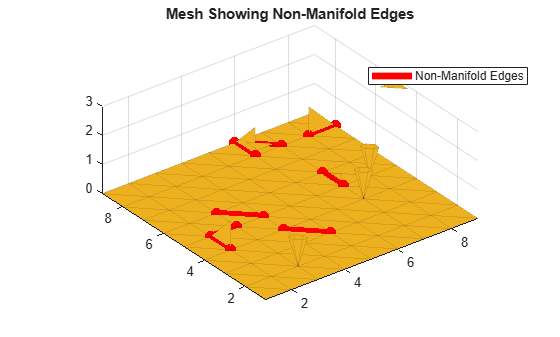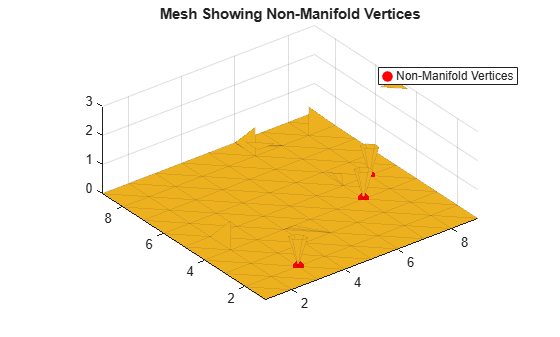# stlFileChecker

Detect and list bad features of STL files

## Description

Use the `stlFileChecker` object to detect and list bad features in STL files. The bad features you can detect using the `stlFileChecker` object are:

• Non-manifold edges

• Non-manifold vertices

• Slivers

• Duplicate vertices

• Normal transition edges

• Free triangles

• T-vertices

## Creation

### Syntax

``obj = stlFileChecker(filename)``
``obj = stlFileChecker(filename,Name=Value)``

### Description

example

````obj = stlFileChecker(filename)` detects bad features in the STL file specified by `filename` and lists the features in the `stlFileChecker` object. ```

example

````obj = stlFileChecker(filename,Name=Value)` sets Input Properties using name-value arguments. For example, `stlFileChecker('s.stl',ShowLog=1)` displays a log of the bad features in file `s.stl`. You can specify multiple name-value arguments. Properties not specified retain their default values.```

### Input Arguments

expand all

Name of the STL file, specified as a character vector or string scalar.

Example: `S = stlFileChecker('s1.stl')`

## Properties

expand all

### Input Properties

Display or hide a log of the bad features in the STL file, specified as `1` or `0`. Specify `1` to display the log of bad features. Specify `0` to hide the log of bad features.

Example: `ShowLog=0`

Data Types: `logical`

Minimum separation between two distinct vertices, specified as a positive scalar in meters. If the distance between two vertices is less than `MinimumSeparation`, then the object considers the vertices as duplicates. The smallest value that you can specify is `2.5e-7`.

Example: `MinimumSeparation=2e-6`

Data Types: `double`

Minimum area of a triangle, specified as a positive scalar in square meters. If the area of a triangle is less than `MinimumArea`, then the object considers the triangle as a sliver. The smallest value that you can specify is `2.5e-12`.

Example: `MinimumArea=3e-10`

Data Types: `double`

### Derived Properties

Edges shared by more than two triangles, returned as a positive scalar.

Data Types: `double`

Vertex connected to two or more surfaces, returned as a positive scalar. A pair of triangles with a common edge belong to the same surface.

Data Types: `double`

Triangle with area less than `MinimumArea`, returned as a positive scalar.

Data Types: `double`

Vertices with separation less than `MinimumSeparation`, returned as a positive scalar.

Data Types: `double`

Edges shared by triangles with normals in opposite directions, returned as a positive scalar.

Data Types: `double`

Triangles with no shared vertex, returned as a positive scalar.

Data Types: `double`

Edge connected to any point other than the end point of a triangle, returned as a positive scalar.

Data Types: `double`

## Examples

collapse all

Use the `stlFileChecker` object to detect and display bad features in an STL file.

```stlFilename = 'sample_stl_file.stl'; s = stlFileChecker(stlFilename)```
```detected 8 non manifold edges detected 3 non manifold vertices detected 0 duplicate Vertices detected 0 Slivers detected 12 Normal Transition Edges detected 2 Free Triangles detected 9 T-Vertices detected 4 duplicate Vertices detected 0 Slivers ```
```s = stlFileChecker with properties: FileName: 'sample_stl_file.stl' Triangulation: [162x3 triangulation] NonManifoldEdges: [8x2 double] NonManifoldVertices: [3x1 double] Slivers: [0x1 double] DuplicateVertices: [4x1 double] NormalTransitionEdges: [12x2 double] FreeTriangles: [2x1 double] TVertices: [9x1 double] ShowLog: 1 MinimumSeparation: 1.0000e-06 MinimumArea: 1.0000e-11 ```

Visualize the non-manifold edges in the STL file.

`showNonManifoldEdges(s)`Visualize the non-manifold vertices in the STL file.

```figure showNonManifoldVertices(s)```Visualize the free triangles in the STL file.

```figure showFreeTriangles(s)```Detect and visualize the T-vertices in an STL file.

`s = stlFileChecker('sample_file.stl',ShowLog=0);`

Visualize the T-vertices in the STL file.

`showTVertices(s)`